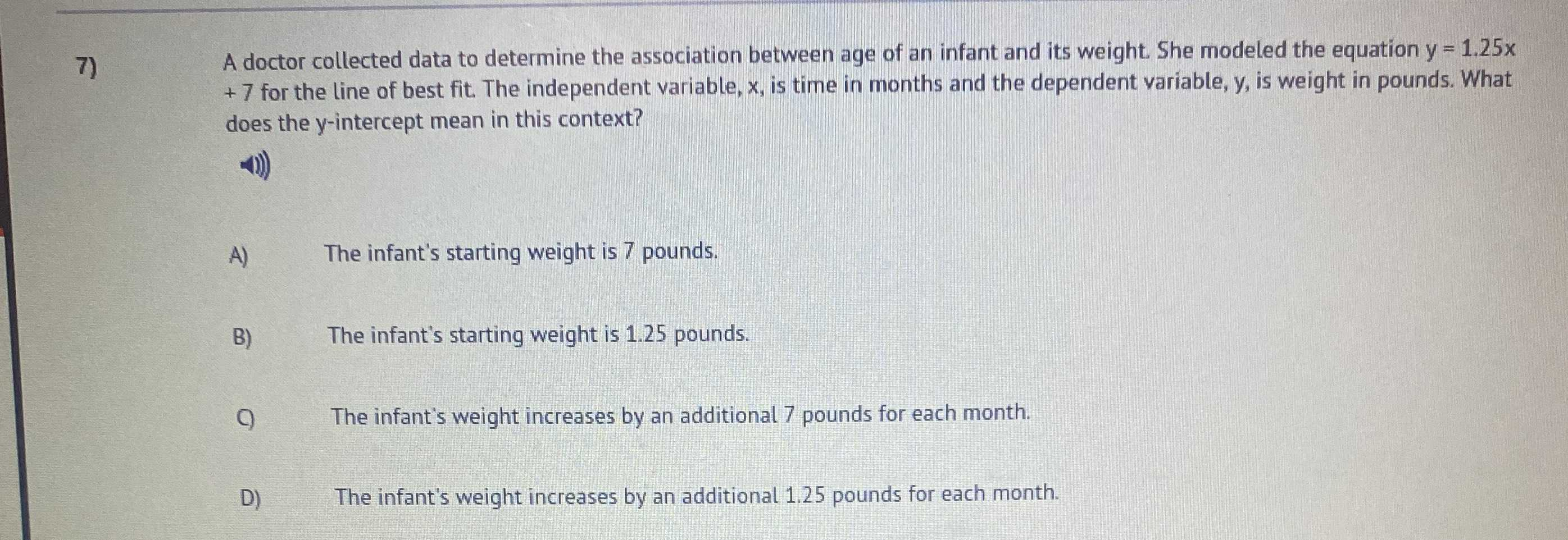### ¿Todavía tienes preguntas de matemáticas?

Pregunte a nuestros tutores expertos
Algebra
PreguntaA doctor collected data to determine the association between age of an infant and its weight. She modeled the equation $$y = 1.25 x$$ $$+ 7$$ for the line of best fit. The independent variable, $$x$$ , is time in months and the dependent variable, $$y$$ , is weight in pounds. What does the $$y$$ -intercept mean in this context?

A) The infant's starting weight is $$7$$ pounds.

B) The infant's starting weight is $$1.25$$ pounds.

C) The infant's weight increases by an additional $$7$$ pounds for each month.

D) The infant's weight increases by an additional $$1.25$$ pounds for each month.